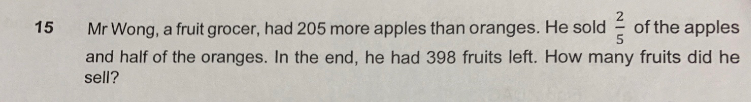# QuestionPlease put in the simplest terms possible so I can teach my child next time, thanks.

Apples    [———u————]

Oranges  [———u————]

Apples sold = (2/5)(u+205) =>  Apples left = (3/5)(u+205) = (6/10) (u+205)

Oranges sold = (1/2)u  => Oranges left = (1/2)u = (5/10)u

(6/10)(u+205) + (5/10)u = 398

6u + 1230 +5u = 3980

11u = 2750

u = 250

Apples sold = (2/5)(u+205) = (2/5) (250+205) = 182

Oranges sold = (1/2)u = (1/2)(250) = 125

Total fruits sold = 182+125 = 307

Let me try with simultaneous equations.

A – O = 205 … (1)

(3/5)A + (1/2)O = 398
A + (5/3)(1/2)O = (5/3)398 … (2)

(2) – (1):
(5/6)O + O = (5/3)398 – 205
(11/6)O = (5/3)398 – 205
O = (6/11)((5/3)398 – 205) = (10/11)398 – (6/11)205 = 361.82 – 111.82 = 250

A = 250 + 205 = 455

So apples sold = (2/5)455 = 182

oranges sold = (1/2)250 = 125

Total fruits sold = 182+125 = 307 #

0 Replies 1 Like